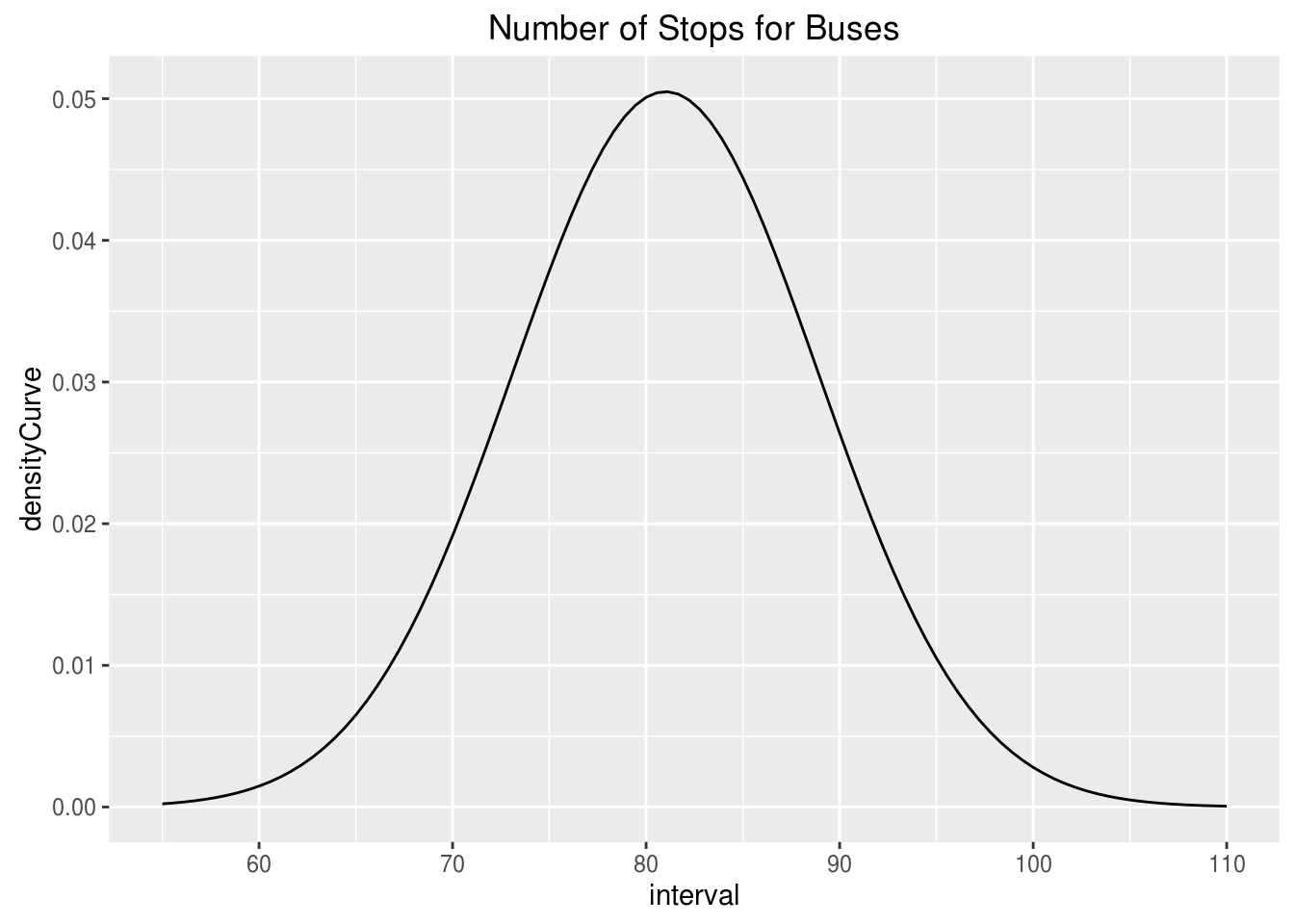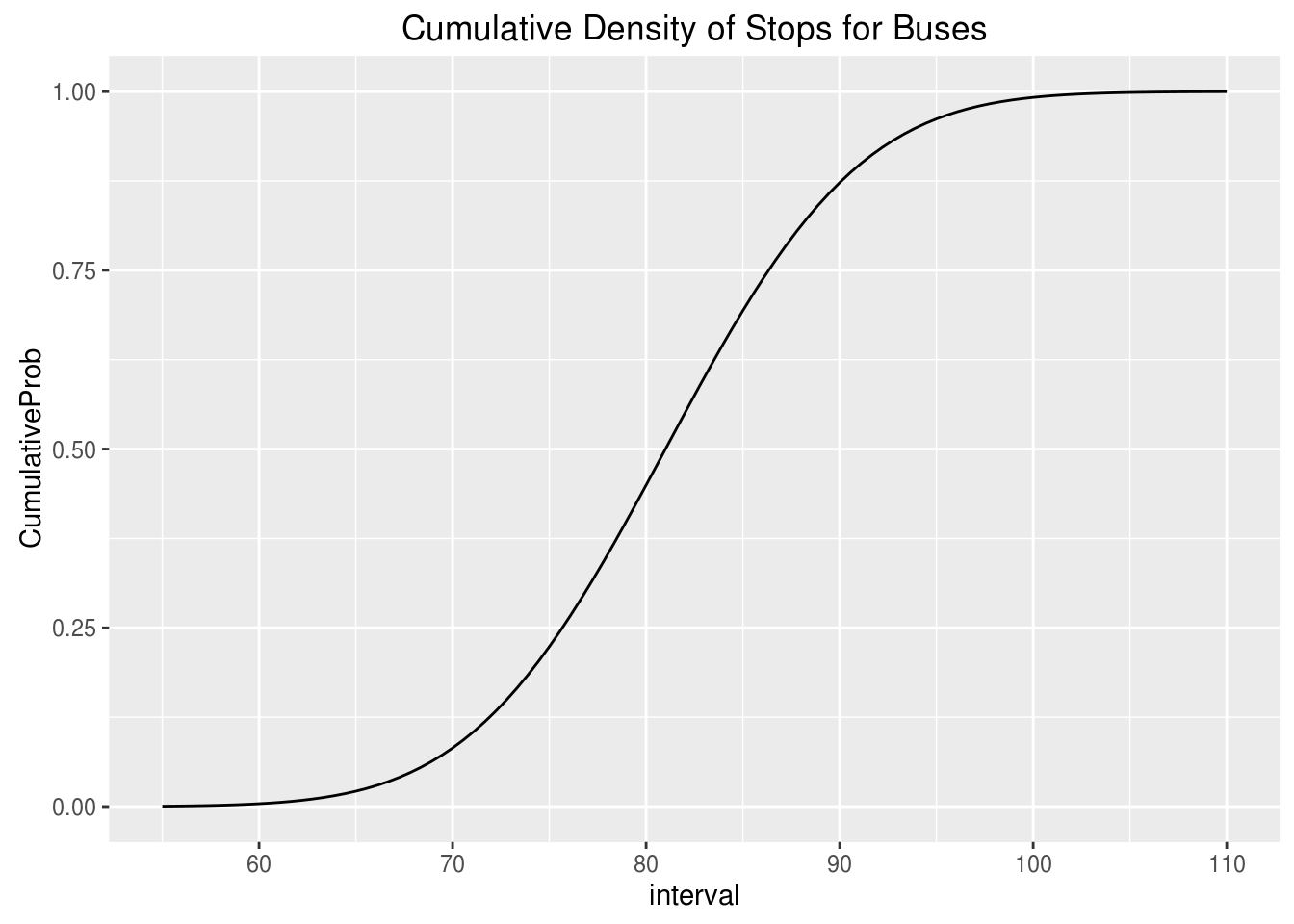# Probability Distribution and Graphs in R

In this post, we will use probability distributions and ggplot2 in R to solve a hypothetical example. This provides a practical example of the use of R in everyday life through the integration of several statistical and coding skills. Below is the scenario.

At a busing company the average number of stops for a bus is 81 with a standard deviation of 7.9. The data is normally distributed. Knowing this complete the following.

• Calculate the interval value to use using the 68-95-99.7 rule
• Calculate the density curve
• Graph the normal curve
• Evaluate the probability of a bus having less then 65 stops
• Evaluate the probability of a bus having more than 93 stops

Calculate the Interval Value

Our first step is to calculate the interval value. This is the range in which 99.7% of the values falls within. Doing this requires knowing the mean and the standard deviation and subtracting/adding the standard deviation as it is multiplied by three from the mean. Below is the code for this.

``````busStopMean<-81
busStopSD<-7.9
busStopMean+3*busStopSD``````
``##  104.7``
``busStopMean-3*busStopSD``
``##  57.3``

The values above mean that we can set are interval between 55 and 110 with 100 buses in the data. Below is the code to set the interval.

``````interval<-seq(55,110, length=100) #length here represents
````100 fictitious buses````

Density Curve

The next step is to calculate the density curve. This is done with our knowledge of the interval, mean, and standard deviation. We also need to use the “dnorm” function. Below is the code for this.

``densityCurve<-dnorm(interval,mean=81,sd=7.9)``

We will now plot the normal curve of our data using ggplot. Before we need to put our “interval” and “densityCurve” variables in a dataframe. We will call the dataframe “normal” and then we will create the plot. Below is the code.

``````library(ggplot2)
normal<-data.frame(interval, densityCurve)
ggplot(normal, aes(interval, densityCurve))+geom_line()+ggtitle("Number of Stops for Buses")``````Probability Calculation

We now want to determine what is the provability of a bus having less than 65 stops. To do this we use the “pnorm” function in R and include the value 65, along with the mean, standard deviation, and tell R we want the lower tail only. Below is the code for completing this.

``pnorm(65,mean = 81,sd=7.9,lower.tail = TRUE)``
``##  0.02141744``

As you can see, at 2% it would be unusually to. We can also plot this using ggplot. First, we need to set a different density curve using the “pnorm” function. Combine this with our “interval” variable in a dataframe and then use this information to make a plot in ggplot2. Below is the code.

``````CumulativeProb<-pnorm(interval, mean=81,sd=7.9,lower.tail = TRUE)
pnormal<-data.frame(interval, CumulativeProb)
ggplot(pnormal, aes(interval, CumulativeProb))+geom_line()+ggtitle("Cumulative Density of Stops for Buses")``````Second Probability Problem

We will now calculate the probability of a bus have 93 or more stops. To make it more interesting we will create a plot that shades the area under the curve for 93 or more stops. The code is a little to complex to explain so just enjoy the visual.

``pnorm(93,mean=81,sd=7.9,lower.tail = FALSE)``
``##  0.06438284``
``````x<-interval
ytop<-dnorm(93,81,7.9)
MyDF<-data.frame(x=x,y=densityCurve)
p<-ggplot(MyDF,aes(x,y))+geom_line()+scale_x_continuous(limits = c(50, 110))
+ggtitle("Probabilty of 93 Stops or More is 6.4%")
shade <- rbind(c(93,0), subset(MyDF, x > 93), c(MyDF[nrow(MyDF), "X"], 0))

p + geom_segment(aes(x=93,y=0,xend=93,yend=ytop)) +